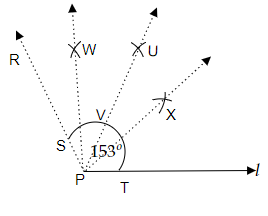# Draw an angle of measure $153^{\circ}$ and divide it into four equal parts.

To do:

We have to draw an angle of measure $153^o$ and divide it into four equal parts.

Solution:Steps of construction:

(i) Let us draw a line $l$ of any length and mark a point $P$ on it.

(ii) Now, by taking a protractor let us place the centre of the protractor on the point $P$ and mark a point $R$ at the measure of angle $153^o$.

(iii) Now, let us join the points $P$ and $R$. Therefore, $\overline{PR}$ has the required angle of measure $153^o$ with the line $l$.

(iv) Now, by taking the compasses with any measure of radius let us draw an arc from point $P$. Let us mark the point of intersection of this arc with the line $l$ and $\overline{PR}$ as points $S$ and $T$ respectively.

(v) Now, by taking compasses with the measure of radius more than half of the length from point $P$ to $R$ let us draw an arc inside the $\overline{PS}$ and mark the point of intersection of this arcs as point $U$. Now, let us join the points $P$ and point $U$.

(vi)  Let us point the point of intersection of $\overline{PU}$ to the arc $ST$ as $V$. Now, by taking the compasses with a radius greater than half of the length from $S$ to $V$ let us draw two arcs and mark the point of intersection to each other as point $W$.

(vii) In a similar way, by taking the compasses with a radius greater than half of the length from $V$ to $T$ let us draw two arcs and mark the point of intersection to each other as point $X$.

(viii) Now, let us join the points $W$ and $P$ and also the points $X$ and $P$ respectively.

(ix) Therefore, $\overline{PX}$,$\overline{PW}$ and $\overline{PU}$ divides the $\overline{PS}$ of angle $153^o$ into four equal parts.